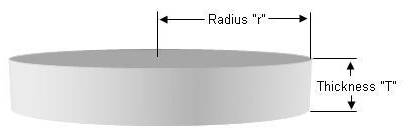Calculating The Amount Of Concrete Needed For A Circular Slab

Calculating the amount of concrete you require for a slab in the shape of a circle can be confusing.Notes:

• Pre-mix concrete comes in bags that are 60 lbs. and 80 lbs.
• A 60 lb. bag provides 0.45 cubic feet of cured concrete.
• A 80 lb. bag provides 0.6 cubic feet of cured concrete.
• If you have concrete delivered it is sold by the yard (which is a cubic yard = 27 cubic feet).

Example:

A circle 6″ thick where the circle has a radius of 5.5' (11' diameter circle)

3.14 x 5.5 x 5.5 x 6 ÷ 12 = 47.5 cubic feet of concrete

47.5 ÷ 0.45 = 106 (105.6) 60 lb bags of concrete

47.5 ÷ 0.6 = 80 (79.2) 80 lb. bags of concrete

47.5 ÷ 27 = 1.8 cubic yards of concrete